# 12.2 The hyperbola  (Page 9/13)

 Page 9 / 13

$\frac{{\left(y-6\right)}^{2}}{36}-\frac{{\left(x+1\right)}^{2}}{16}=1$

$\frac{{\left(x-2\right)}^{2}}{49}-\frac{{\left(y+7\right)}^{2}}{49}=1$

$\frac{{\left(x-2\right)}^{2}}{{7}^{2}}-\frac{{\left(y+7\right)}^{2}}{{7}^{2}}=1;\text{\hspace{0.17em}}$ vertices: $\text{\hspace{0.17em}}\left(9,-7\right),\left(-5,-7\right);\text{\hspace{0.17em}}$ foci: $\text{\hspace{0.17em}}\left(2+7\sqrt{2},-7\right),\left(2-7\sqrt{2},-7\right);\text{\hspace{0.17em}}$ asymptotes: $\text{\hspace{0.17em}}y=x-9,y=-x-5$

$4{x}^{2}-8x-9{y}^{2}-72y+112=0$

$-9{x}^{2}-54x+9{y}^{2}-54y+81=0$

$\frac{{\left(x+3\right)}^{2}}{{3}^{2}}-\frac{{\left(y-3\right)}^{2}}{{3}^{2}}=1;\text{\hspace{0.17em}}$ vertices: $\text{\hspace{0.17em}}\left(0,3\right),\left(-6,3\right);\text{\hspace{0.17em}}$ foci: $\text{\hspace{0.17em}}\left(-3+3\sqrt{2},1\right),\left(-3-3\sqrt{2},1\right);\text{\hspace{0.17em}}$ asymptotes: $\text{\hspace{0.17em}}y=x+6,y=-x$

$4{x}^{2}-24x-36{y}^{2}-360y+864=0$

$-4{x}^{2}+24x+16{y}^{2}-128y+156=0$

$\frac{{\left(y-4\right)}^{2}}{{2}^{2}}-\frac{{\left(x-3\right)}^{2}}{{4}^{2}}=1;\text{\hspace{0.17em}}$ vertices: $\text{\hspace{0.17em}}\left(3,6\right),\left(3,2\right);\text{\hspace{0.17em}}$ foci: $\text{\hspace{0.17em}}\left(3,4+2\sqrt{5}\right),\left(3,4-2\sqrt{5}\right);\text{\hspace{0.17em}}$ asymptotes: $\text{\hspace{0.17em}}y=\frac{1}{2}\left(x-3\right)+4,y=-\frac{1}{2}\left(x-3\right)+4$

$-4{x}^{2}+40x+25{y}^{2}-100y+100=0$

${x}^{2}+2x-100{y}^{2}-1000y+2401=0$

$\frac{{\left(y+5\right)}^{2}}{{7}^{2}}-\frac{{\left(x+1\right)}^{2}}{{70}^{2}}=1;\text{\hspace{0.17em}}$ vertices: $\text{\hspace{0.17em}}\left(-1,2\right),\left(-1,-12\right);\text{\hspace{0.17em}}$ foci: $\text{\hspace{0.17em}}\left(-1,-5+7\sqrt{101}\right),\left(-1,-5-7\sqrt{101}\right);\text{\hspace{0.17em}}$ asymptotes: $\text{\hspace{0.17em}}y=\frac{1}{10}\left(x+1\right)-5,y=-\frac{1}{10}\left(x+1\right)-5$

$-9{x}^{2}+72x+16{y}^{2}+16y+4=0$

$4{x}^{2}+24x-25{y}^{2}+200y-464=0$

$\frac{{\left(x+3\right)}^{2}}{{5}^{2}}-\frac{{\left(y-4\right)}^{2}}{{2}^{2}}=1;\text{\hspace{0.17em}}$ vertices: $\text{\hspace{0.17em}}\left(2,4\right),\left(-8,4\right);\text{\hspace{0.17em}}$ foci: $\text{\hspace{0.17em}}\left(-3+\sqrt{29},4\right),\left(-3-\sqrt{29},4\right);\text{\hspace{0.17em}}$ asymptotes: $\text{\hspace{0.17em}}y=\frac{2}{5}\left(x+3\right)+4,y=-\frac{2}{5}\left(x+3\right)+4$

For the following exercises, find the equations of the asymptotes for each hyperbola.

$\frac{{y}^{2}}{{3}^{2}}-\frac{{x}^{2}}{{3}^{2}}=1$

$\frac{{\left(x-3\right)}^{2}}{{5}^{2}}-\frac{{\left(y+4\right)}^{2}}{{2}^{2}}=1$

$y=\frac{2}{5}\left(x-3\right)-4,y=-\frac{2}{5}\left(x-3\right)-4$

$\frac{{\left(y-3\right)}^{2}}{{3}^{2}}-\frac{{\left(x+5\right)}^{2}}{{6}^{2}}=1$

$9{x}^{2}-18x-16{y}^{2}+32y-151=0$

$y=\frac{3}{4}\left(x-1\right)+1,y=-\frac{3}{4}\left(x-1\right)+1$

$16{y}^{2}+96y-4{x}^{2}+16x+112=0$

## Graphical

For the following exercises, sketch a graph of the hyperbola, labeling vertices and foci.

$\frac{{x}^{2}}{49}-\frac{{y}^{2}}{16}=1$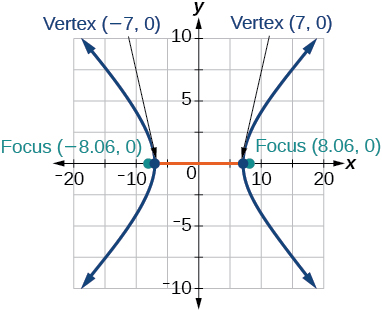$\frac{{x}^{2}}{64}-\frac{{y}^{2}}{4}=1$

$\frac{{y}^{2}}{9}-\frac{{x}^{2}}{25}=1$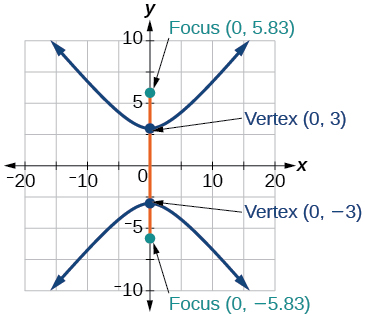$81{x}^{2}-9{y}^{2}=1$

$\frac{{\left(y+5\right)}^{2}}{9}-\frac{{\left(x-4\right)}^{2}}{25}=1$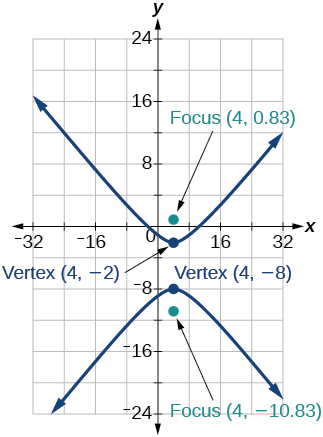$\frac{{\left(x-2\right)}^{2}}{8}-\frac{{\left(y+3\right)}^{2}}{27}=1$

$\frac{{\left(y-3\right)}^{2}}{9}-\frac{{\left(x-3\right)}^{2}}{9}=1$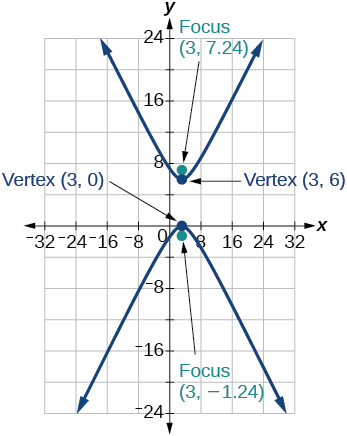$-4{x}^{2}-8x+16{y}^{2}-32y-52=0$

${x}^{2}-8x-25{y}^{2}-100y-109=0$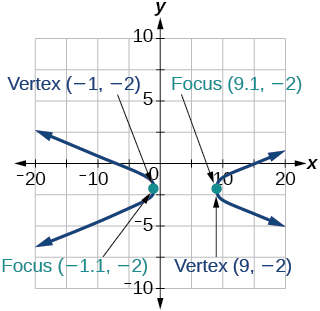$-{x}^{2}+8x+4{y}^{2}-40y+88=0$

$64{x}^{2}+128x-9{y}^{2}-72y-656=0$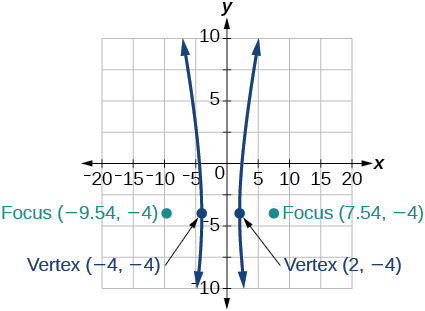$16{x}^{2}+64x-4{y}^{2}-8y-4=0$

$-100{x}^{2}+1000x+{y}^{2}-10y-2575=0$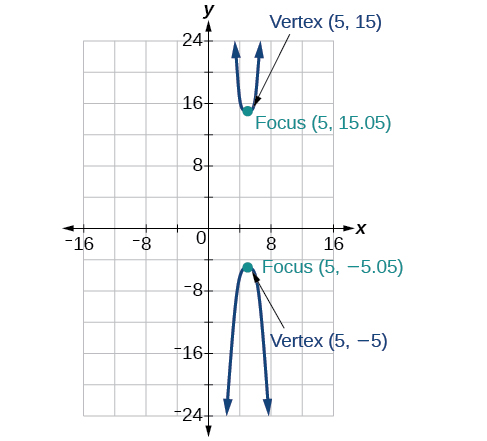$4{x}^{2}+16x-4{y}^{2}+16y+16=0$

For the following exercises, given information about the graph of the hyperbola, find its equation.

Vertices at $\text{\hspace{0.17em}}\left(3,0\right)\text{\hspace{0.17em}}$ and $\text{\hspace{0.17em}}\left(-3,0\right)\text{\hspace{0.17em}}$ and one focus at $\text{\hspace{0.17em}}\left(5,0\right).$

$\frac{{x}^{2}}{9}-\frac{{y}^{2}}{16}=1$

Vertices at $\text{\hspace{0.17em}}\left(0,6\right)\text{\hspace{0.17em}}$ and $\text{\hspace{0.17em}}\left(0,-6\right)\text{\hspace{0.17em}}$ and one focus at $\text{\hspace{0.17em}}\left(0,-8\right).$

Vertices at $\text{\hspace{0.17em}}\left(1,1\right)\text{\hspace{0.17em}}$ and $\text{\hspace{0.17em}}\left(11,1\right)\text{\hspace{0.17em}}$ and one focus at $\text{\hspace{0.17em}}\left(12,1\right).$

$\frac{{\left(x-6\right)}^{2}}{25}-\frac{{\left(y-1\right)}^{2}}{11}=1$

Center: $\text{\hspace{0.17em}}\left(0,0\right);$ vertex: $\text{\hspace{0.17em}}\left(0,-13\right);$ one focus: $\text{\hspace{0.17em}}\left(0,\sqrt{313}\right).$

Center: $\text{\hspace{0.17em}}\left(4,2\right);$ vertex: $\text{\hspace{0.17em}}\left(9,2\right);$ one focus: $\text{\hspace{0.17em}}\left(4+\sqrt{26},2\right).$

$\frac{{\left(x-4\right)}^{2}}{25}-\frac{{\left(y-2\right)}^{2}}{1}=1$

Center: $\text{\hspace{0.17em}}\left(3,5\right);\text{\hspace{0.17em}}$ vertex: $\text{\hspace{0.17em}}\left(3,11\right);\text{\hspace{0.17em}}$ one focus: $\text{\hspace{0.17em}}\left(3,5+2\sqrt{10}\right).$

For the following exercises, given the graph of the hyperbola, find its equation.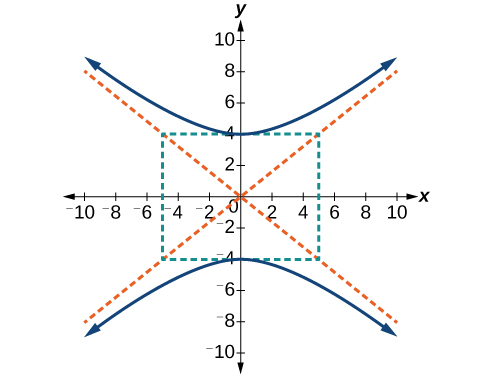$\frac{{y}^{2}}{16}-\frac{{x}^{2}}{25}=1$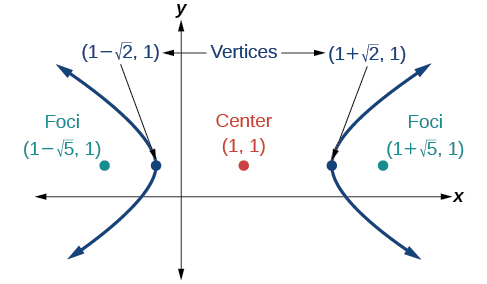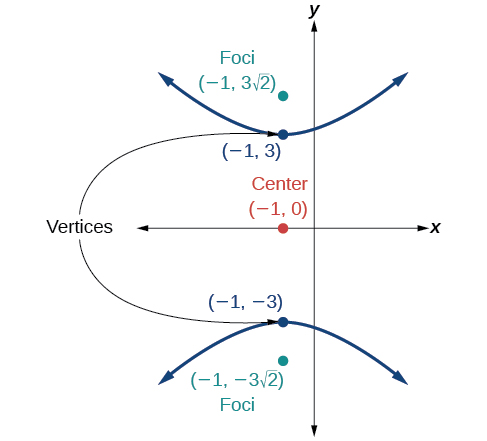$\frac{{y}^{2}}{9}-\frac{{\left(x+1\right)}^{2}}{9}=1$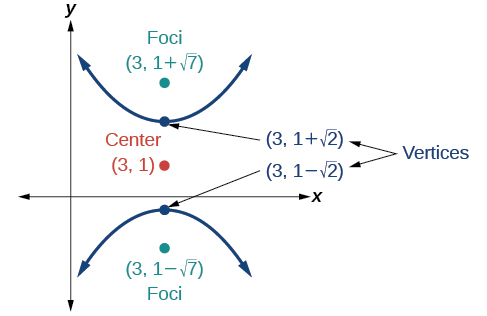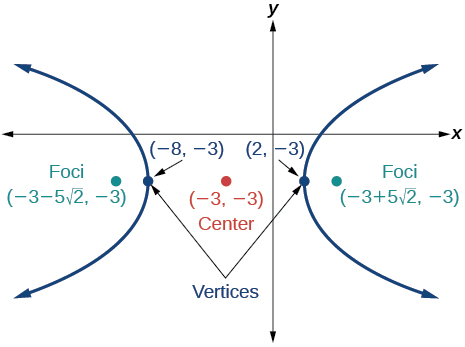$\frac{{\left(x+3\right)}^{2}}{25}-\frac{{\left(y+3\right)}^{2}}{25}=1$

## Extensions

For the following exercises, express the equation for the hyperbola as two functions, with $\text{\hspace{0.17em}}y\text{\hspace{0.17em}}$ as a function of $\text{\hspace{0.17em}}x.\text{\hspace{0.17em}}$ Express as simply as possible. Use a graphing calculator to sketch the graph of the two functions on the same axes.

$\frac{{x}^{2}}{4}-\frac{{y}^{2}}{9}=1$

$\frac{{y}^{2}}{9}-\frac{{x}^{2}}{1}=1$

$y\left(x\right)=3\sqrt{{x}^{2}+1},y\left(x\right)=-3\sqrt{{x}^{2}+1}$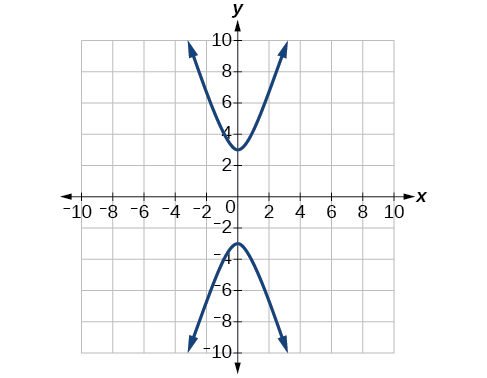$\frac{{\left(x-2\right)}^{2}}{16}-\frac{{\left(y+3\right)}^{2}}{25}=1$

$-4{x}^{2}-16x+{y}^{2}-2y-19=0$

$y\left(x\right)=1+2\sqrt{{x}^{2}+4x+5},y\left(x\right)=1-2\sqrt{{x}^{2}+4x+5}$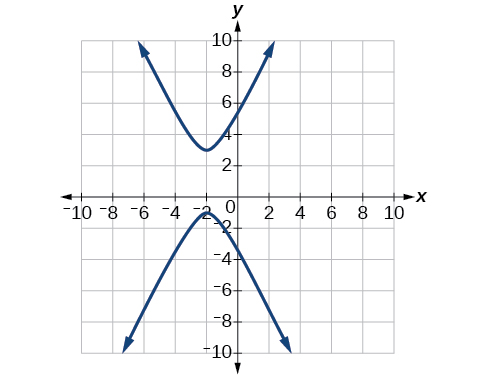$4{x}^{2}-24x-{y}^{2}-4y+16=0$

## Real-world applications

For the following exercises, a hedge is to be constructed in the shape of a hyperbola near a fountain at the center of the yard. Find the equation of the hyperbola and sketch the graph.

The hedge will follow the asymptotes and its closest distance to the center fountain is 5 yards.

$\frac{{x}^{2}}{25}-\frac{{y}^{2}}{25}=1$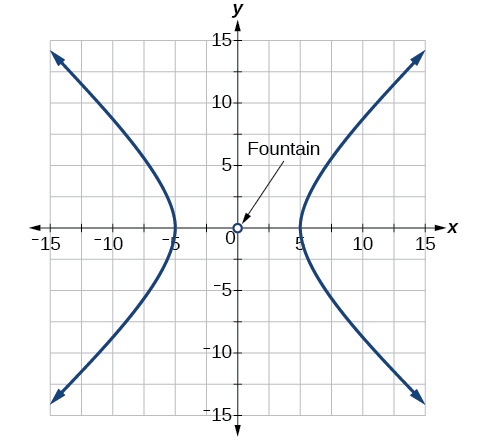The hedge will follow the asymptotes and its closest distance to the center fountain is 6 yards.

The hedge will follow the asymptotes $\text{\hspace{0.17em}}y=\frac{1}{2}x\text{\hspace{0.17em}}$ and $\text{\hspace{0.17em}}y=-\frac{1}{2}x,$ and its closest distance to the center fountain is 10 yards.

$\frac{{x}^{2}}{100}-\frac{{y}^{2}}{25}=1$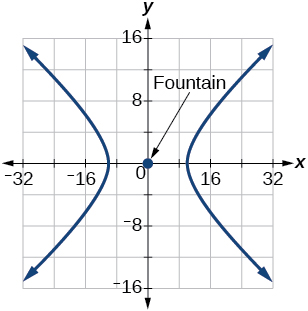The hedge will follow the asymptotes $\text{\hspace{0.17em}}y=\frac{2}{3}x\text{\hspace{0.17em}}$ and $\text{\hspace{0.17em}}y=-\frac{2}{3}x,$ and its closest distance to the center fountain is 12 yards.

The hedge will follow the asymptotes and its closest distance to the center fountain is 20 yards.

$\frac{{x}^{2}}{400}-\frac{{y}^{2}}{225}=1$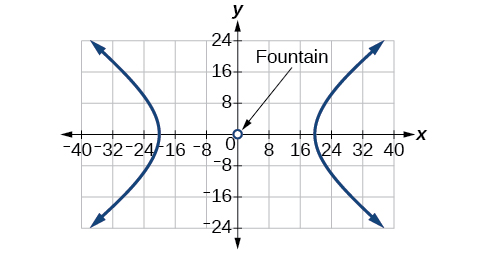For the following exercises, assume an object enters our solar system and we want to graph its path on a coordinate system with the sun at the origin and the x-axis as the axis of symmetry for the object's path. Give the equation of the flight path of each object using the given information.

The object enters along a path approximated by the line $\text{\hspace{0.17em}}y=x-2\text{\hspace{0.17em}}$ and passes within 1 au (astronomical unit) of the sun at its closest approach, so that the sun is one focus of the hyperbola. It then departs the solar system along a path approximated by the line $\text{\hspace{0.17em}}y=-x+2.\text{\hspace{0.17em}}$

The object enters along a path approximated by the line $\text{\hspace{0.17em}}y=2x-2\text{\hspace{0.17em}}$ and passes within 0.5 au of the sun at its closest approach, so the sun is one focus of the hyperbola. It then departs the solar system along a path approximated by the line $\text{\hspace{0.17em}}y=-2x+2.\text{\hspace{0.17em}}$

$\frac{{\left(x-1\right)}^{2}}{0.25}-\frac{{y}^{2}}{0.75}=1$

The object enters along a path approximated by the line $\text{\hspace{0.17em}}y=0.5x+2\text{\hspace{0.17em}}$ and passes within 1 au of the sun at its closest approach, so the sun is one focus of the hyperbola. It then departs the solar system along a path approximated by the line $\text{\hspace{0.17em}}y=-0.5x-2.\text{\hspace{0.17em}}$

The object enters along a path approximated by the line $\text{\hspace{0.17em}}y=\frac{1}{3}x-1\text{\hspace{0.17em}}$ and passes within 1 au of the sun at its closest approach, so the sun is one focus of the hyperbola. It then departs the solar system along a path approximated by the line

$\frac{{\left(x-3\right)}^{2}}{4}-\frac{{y}^{2}}{5}=1$

The object It enters along a path approximated by the line $\text{\hspace{0.17em}}y=3x-9\text{\hspace{0.17em}}$ and passes within 1 au of the sun at its closest approach, so the sun is one focus of the hyperbola. It then departs the solar system along a path approximated by the line $\text{\hspace{0.17em}}y=-3x+9.\text{\hspace{0.17em}}$

sinx sin2x is linearly dependent
what is a reciprocal
The reciprocal of a number is 1 divided by a number. eg the reciprocal of 10 is 1/10 which is 0.1
Shemmy
Reciprocal is a pair of numbers that, when multiplied together, equal to 1. Example; the reciprocal of 3 is ⅓, because 3 multiplied by ⅓ is equal to 1
Jeza
each term in a sequence below is five times the previous term what is the eighth term in the sequence
I don't understand how radicals works pls
How look for the general solution of a trig function
stock therom F=(x2+y2) i-2xy J jaha x=a y=o y=b
sinx sin2x is linearly dependent
cr
root under 3-root under 2 by 5 y square
The sum of the first n terms of a certain series is 2^n-1, Show that , this series is Geometric and Find the formula of the n^th
cosA\1+sinA=secA-tanA
Wrong question
why two x + seven is equal to nineteen.
The numbers cannot be combined with the x
Othman
2x + 7 =19
humberto
2x +7=19. 2x=19 - 7 2x=12 x=6
Yvonne
because x is 6
SAIDI
what is the best practice that will address the issue on this topic? anyone who can help me. i'm working on my action research.
simplify each radical by removing as many factors as possible (a) √75
how is infinity bidder from undefined?
what is the value of x in 4x-2+3
give the complete question
Shanky
4x=3-2 4x=1 x=1+4 x=5 5x
Olaiya
hi can you give another equation I'd like to solve it
Daniel
what is the value of x in 4x-2+3
Olaiya
if 4x-2+3 = 0 then 4x = 2-3 4x = -1 x = -(1÷4) is the answer.
Jacob
4x-2+3 4x=-3+2 4×=-1 4×/4=-1/4
LUTHO
then x=-1/4
LUTHO
4x-2+3 4x=-3+2 4x=-1 4x÷4=-1÷4 x=-1÷4
LUTHO
A research student is working with a culture of bacteria that doubles in size every twenty minutes. The initial population count was  1350  bacteria. Rounding to five significant digits, write an exponential equation representing this situation. To the nearest whole number, what is the population size after  3  hours?
f(x)= 1350. 2^(t/20); where t is in hours.
MerkebByBy Sandy YamaneBy Brooke DelaneyBy Brooke DelaneyBy Madison ChristianBy Yasser IbrahimBy OpenStaxBy Jordon HumphreysBy Christine ZeelieBy OpenStaxBy Robert Murphy